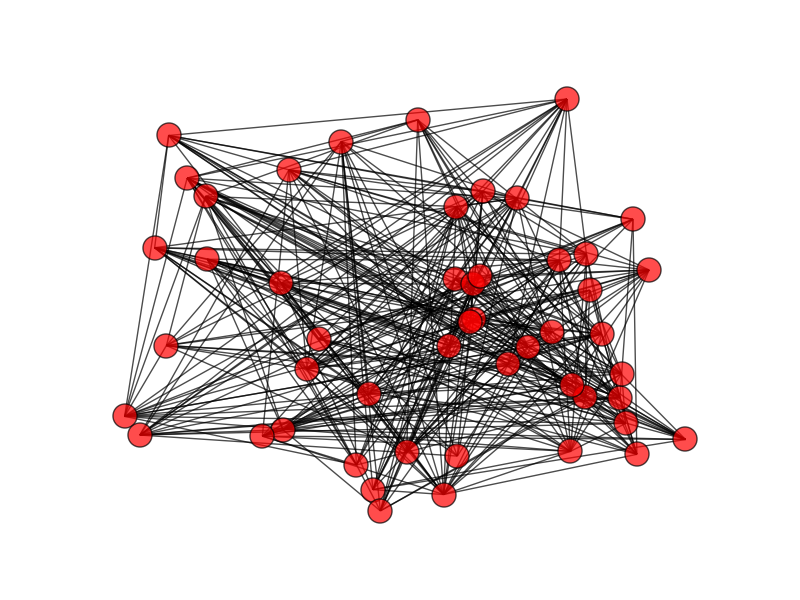# Bounding a graph’s diameter by its spectrum

You can get upper and lower bounds on the diameter of a connected graph G from its spectrum.

If G has r distinct eigenvalues—whether of the adjacency matrix A, Laplacian L, or signless Laplacian Q—then the its diameter d is at most r-1. (Definitions of these matrices here.)

If G has n vertices then we have an lower bound on the diameter: d ≥ 4/nλ2 where λ2 is the algebraic connectivity, the second eigenvalue of the graph Laplacian.

Let Δ be the maximum degree of G. Then we also have the following upper bound:How well do these bounds work? Let’s look at a random graph with 50 vertices.The diameter of this graph is 3. The highest vertex degree is 22.

Each of the three matrices A, L, and Q have 50 distinct eigenvalues. So that says the diameter should be less than 49, which it certainly is.

The lower bound based on algebraic connectivity is 0.0129, and the upper bound is 32.

Altogether, we estimated the diameter, whose true value is 3, to be between 0.0129 and 32. Which is correct, but not very tight.

Maybe the bounds weren’t very good because the diameter was small. Let’s look at graph with a much bigger diameter, a circle with 50 nodes.Now this graph has diameter 25. The maximum node degree is 2, because every node has degree 2.

Each of the three matrices A, L, and Q have 26 distinct eigenvalues, which predicts that the graph diameter will be no more than 25. In this case, the upper bound is exact.

The algebraic connectivity gives a lower bound of 5.0727 for the diameter and an upper bound of 180. So while these bounds are correct, they are not especially tight either.

Source: Handbook of Linear Algebra

## 3 thoughts on “Bounding a graph’s diameter by its spectrum”

1. Thanks!

2. It appears more of an ellipse than a circle, like that which would mimic the current Milky-way. In Euclidean geometry, we have an edge, a face, and a vertex to work with.